The STDRATE Procedure

Rate

Subsections:

A major task in epidemiology is to compare event frequencies for groups of people. Both rate and risk are commonly used to measure event frequency in the comparison. Rate is a measure of change in one quantity per unit of another quantity. An event rate measures how fast the events are occurring. In contrast, an event risk is the probability that an event occurs over a specified follow-up time period.

An event rate of a population over a specified time period can be defined as the number of new events divided by the population-time of the population over the same time period,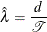where d is the number of events and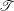is the population-time that is computed by adding up the time contributed by each subject in the population over the specified time period.

For a general population, the subsets (strata) might not be homogeneous enough to have a similar rate. Thus, the rate for each stratum should be computed separately to reflect this discrepancy. For a population that consists of K homogeneous strata (such as different age groups), the stratum-specific rate for the jth stratum in a population is computed as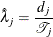where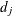is the number of events and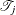is the population-time for subjects in the jth stratum of the population.

Assuming the number of events in the jth stratum,, has a Poisson distribution, the variance of
is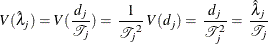By using the method of statistical differentials (Elandt-Johnson and Johnson 1980, pp. 70–71), the variance of the logarithm of rate can be estimated by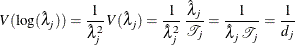Because the rate value can be very small, especially for rare events, it is sometimes expressed in terms of the product of a multiplier and the rate itself. For example, a rate can be expressed as the number of events per 100,000 person-years.

Normal Distribution Confidence Interval for Rate

A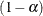confidence interval for
based on a normal distribution is given by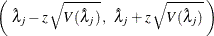where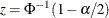is the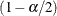quantile of the standard normal distribution.

Lognormal Distribution Confidence Interval for Rate

Aconfidence interval for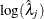based on a normal distribution is given by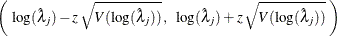whereis thequantile of the standard normal distribution and the variance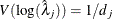.

Thus, aconfidence interval forbased on a lognormal distribution is given by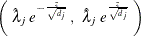Poisson Distribution Confidence Interval for Rate

Denote the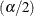quantile for the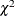distribution with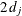degrees of freedom by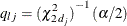Denote thequantiles for thedistribution with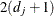degrees of freedom by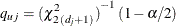Then aconfidence interval forbased on thedistribution is given by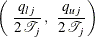Confidence Interval for Rate Difference Statistic

For rate estimates from two independent samples,
and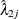, aconfidence interval for the rate difference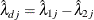is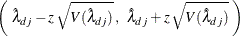whereis thequantile of the standard normal distribution and the variance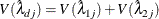Confidence Interval for Rate Ratio Statistic

For rate estimates from two independent samples,and, aconfidence interval for the log rate ratio statistic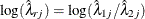is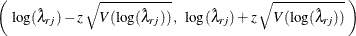whereis thequantile of the standard normal distribution and the variance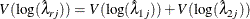Thus, aconfidence interval for the rate ratio statistic
is given by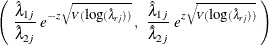Confidence Interval for Rate SMR

At stratum j, a stratum-specific standardized morbidity/mortality ratio is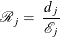where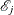is the expected number of events.

With the rateSMR can be expressed as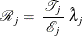Thus, aconfidence interval for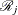is given by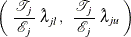where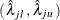is aconfidence interval for the rate.